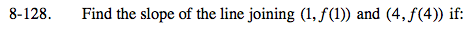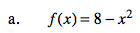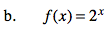### Home > PC > Chapter 8 > Lesson 8.2.4 > Problem8-128

8-128.
1. Find the slope of the line joining (1, f (1)) and (4, f (4)) if: Homework Help ✎

1. f(x) = 8 − x2

2. f(x) = 2x